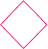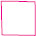# User ForumSubject :IMO    Class : Class 4

If+++= 1240 and 1000 -=, then the value of-=?

A 1000
B 690
C 380
D 310

actually I thought that we should add 310 and 690.

Class : Class 4
Option C 380

Class : Class 8
380

## Ans 3:

Class : Class 5
Answer is C as the question says that 4 diamonds is 1240,we have to divide to get a diamond's value, which is 1240 divided by 4, the answer comes as 310. If we subtract a diamond from 1000, which is 1000-310, the answer comes as a square, which is 690. If we subtract a diamond from a

Class : Class 4
C

## Ans 5:

Class : Class 5
The answer is C as the question says that 4 diamonds is 1240 and 1240 divided by 4 is 310 and if we subtract a diamond from 1000 which means 1000-310,the answer comes as one square which is 690 and if we subtract a diamond from a square which means 690-310,the answer comes as 380.